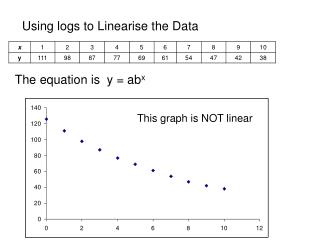DownloadDownload PresentationUsing logs to Linearise the Data

# Using logs to Linearise the Data

Download Presentation## Using logs to Linearise the Data

- - - - - - - - - - - - - - - - - - - - - - - - - - - E N D - - - - - - - - - - - - - - - - - - - - - - - - - - -
##### Presentation Transcript

1. Using logs to Linearise the Data The equation is y = abx This graph is NOT linear

2. Using logs to Linearise the Data The equation is y = abx log y = log(abx) log y = log a + logbxUsing the addition rule log(AB) = logA + logB log y = log a + (xlogb)Using the drop down infront rule log y = (logb) x + logaRearranging to match with y = mx + c Take logs of both sides

3. log y = (logb)x + logaRearranging to match with y=mx + c Matching up : Y axis = log y gradient =m = logb x axis = x C = log a So make a new table of values x = x Y = logy

4. Plot x values on the x axis and logy values on the y axis

5. The equation of the line is y = -0.0522x + 2.0973 Y m X c log y = logb x + loga Matching up : y = mx + c gradient = log b = -0.0522 C = log a = 2.0973

6. gradient = log b = -0.0522 To find b do forwards and back b  log it = –0.0522 Backwards –0.0522  10 it  b b = 10–0.0522 = 0.8867

7. y intercept = log a = 2.0973 To find a do forwards and back a  log it = 2.0973 Backwards 2.0973  10 it  a a = 102.0973 = 125.1

8. The exponential equation is y = abx y = 125.1×0.887x

9. Using the equation y = 125.1×0.887x If x = 5.5 find y y = 125.1×0.8875.5 = 64.7 Check if the answer is consistent with the table x = 5.5 find y y = 64.7 which is consistent with the table

10. Using the equation y = 125.1×0.887x If y = 65 find x 65 = 125.1×0.887x Take logs of both sides log65 = log(125.1×0.887x) = log(125.1)+log(0.887x) = log(125.1)+xlog(0.887) Using the addition rulelog(AB) = logA + logB Using the drop down infront rule

11. log 65 = log(125.1)+xlog(0.887) Forwards and backwards x ×log0.887  +log 125.1 = log65 log65 –log 125.1  ÷log0.887 = x x = 5.46 Check if the answer is consistent with the table y = 65 find x x = 5.46 which is consistent with the table